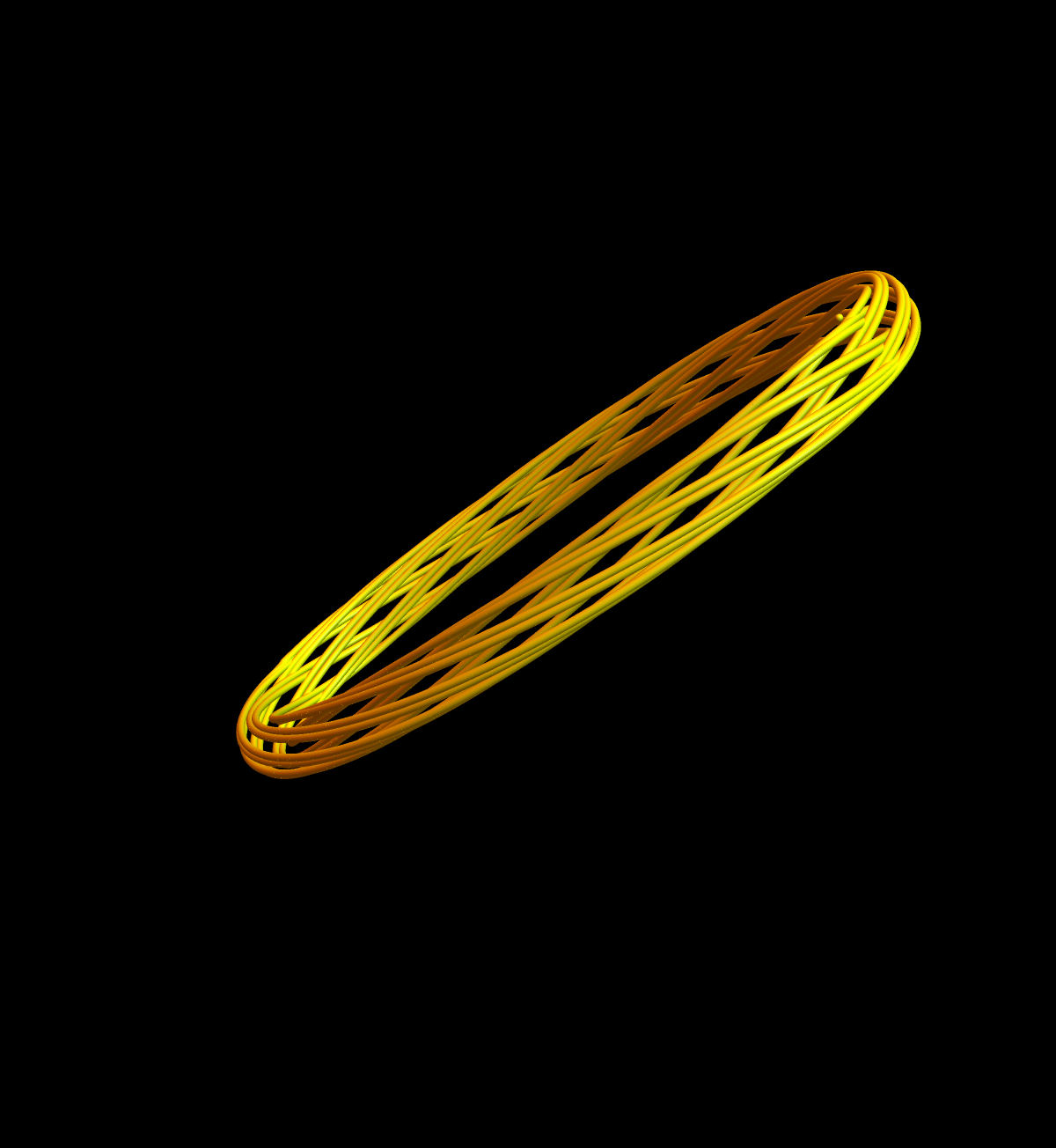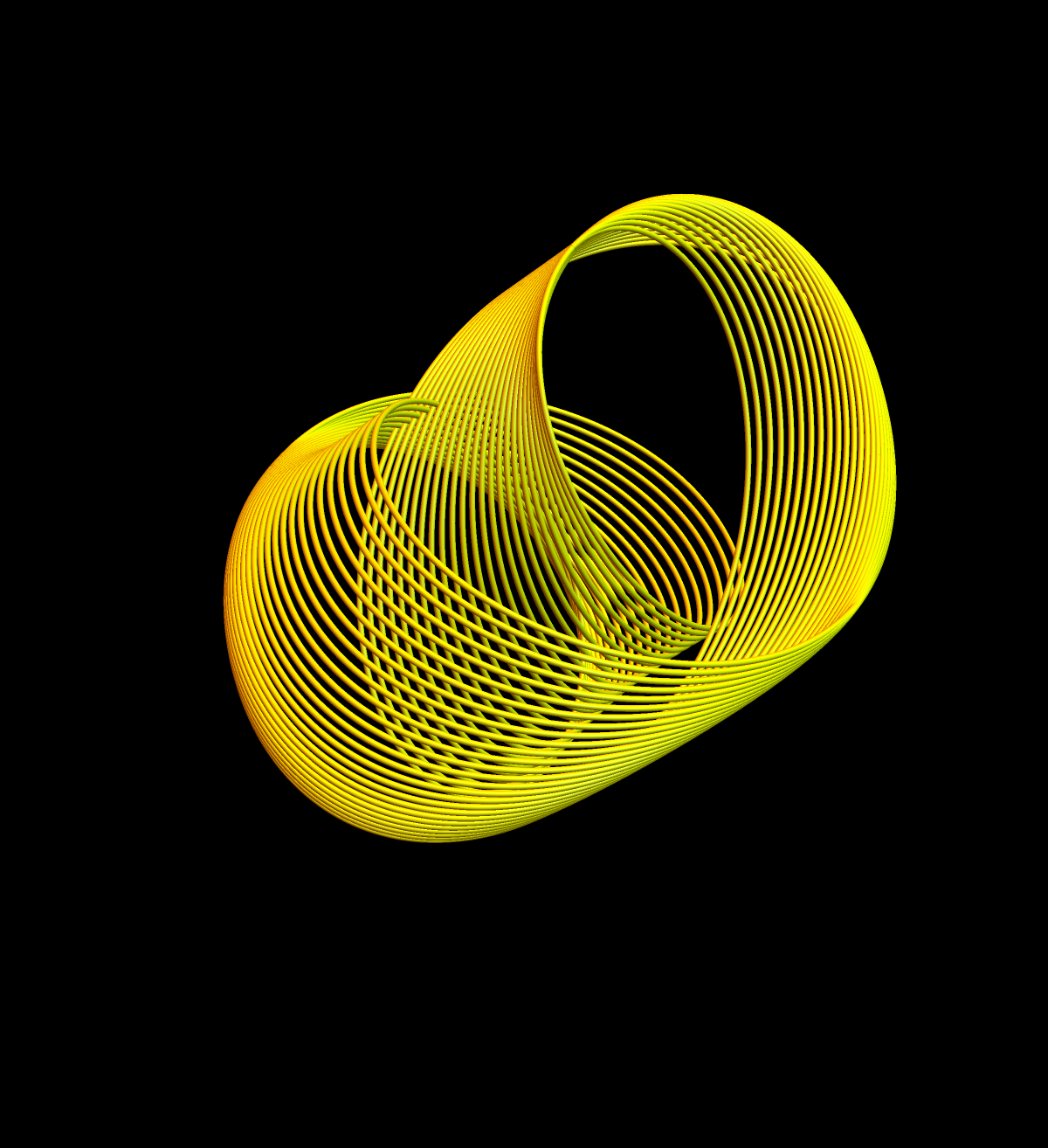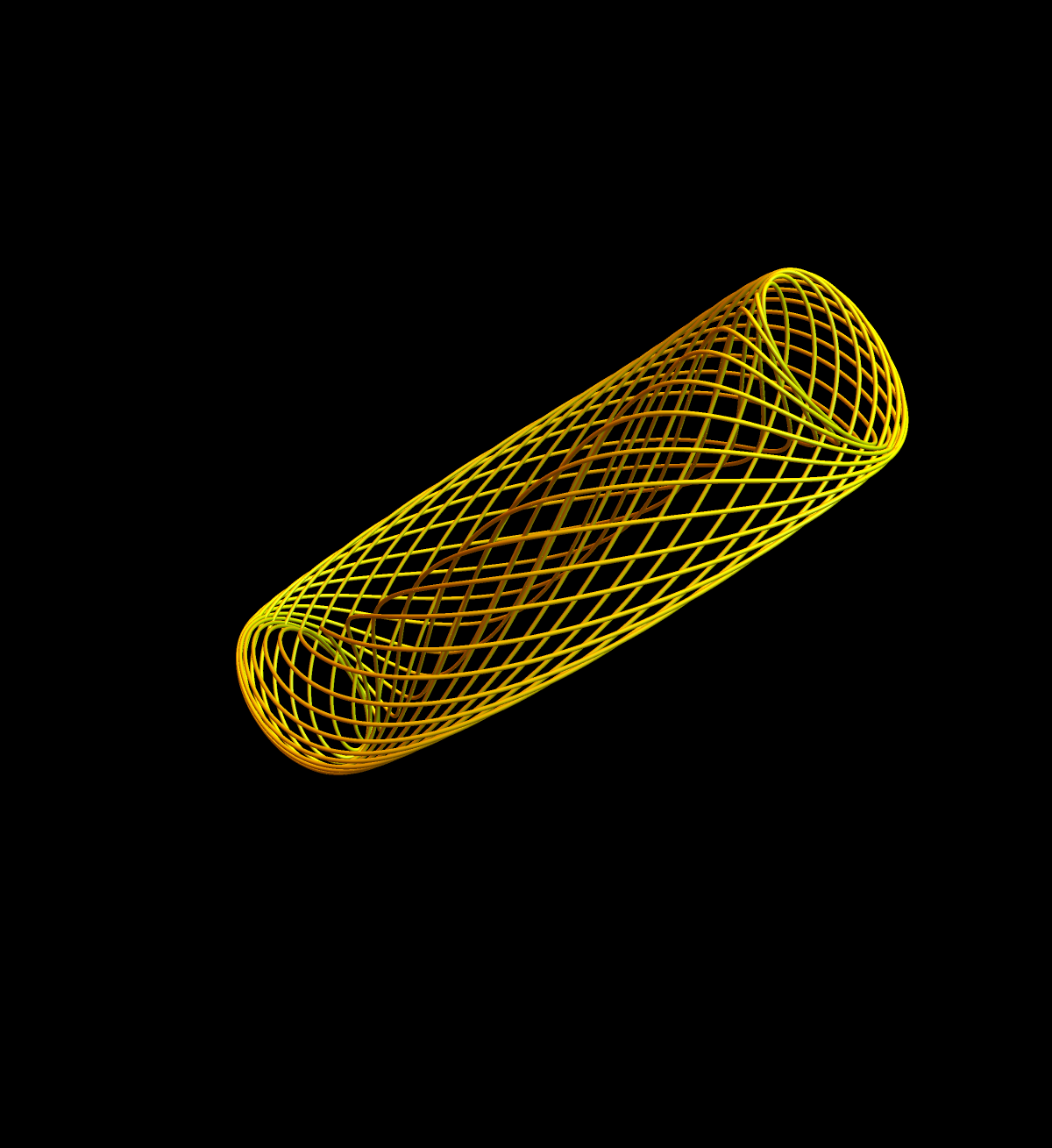# Partial differential equations on graphs

This project with Annie Rak started in the summer 2016 as a HCRP project. The topic is ``differential equations on graphs". We explored in the summer 2016 first various dynamical systems on networks. The topic of discrete PDEs allows for expository, experimental as well as theoretical investigations in an area of mathematics which links discrete mathematics with dynamical system theory and discrete stochastic processes (as PDEs related to the Laplacian are always closely related to diffusion processes). Here are some topics we played with:
• Heat and wave equations u'=-Lu, u''=-Lu
• Linear and nonlinear ave equations like u''=-Lu+sin(u)
• Geometric evolution in the geometry of graphs like PDEs on forms
• Transport and advection consensus dynamics like u'=-L+u on a directed graph.
• Burger and fluid like equations u'= T(u,u) with quadratic difference operator
• Integrability, like Sine-Gordon which generalizes Volterra-Toda like systems.
Annie got HCRP funding also for the Fall of 2016 and used the topic for her senior thesis (which will be submitted in November 2016). I will add a bit more about Annie's specific work later, once the thesis is finished. It is more focused on advection dynamics and its relation with discrete stochastic processes defined on the graph.
 Direct Media Links: Webm, Ogg Ipod, Quicktime. Direct Media Links: Webm, Ogg Ipod, Quicktime.
The following pictures show are some trajectories of the Sine-Gordon equation
u'' = -L u + sin(u)
on the graph K2, where the energy surface is three dimensional and appears to be foliated by two-dimensional tori:The system appears to be integrable. It is a Hamiltonian system in a four dimensional phase space with a three dimensional energy surface. The orbits appear to be located on two dimensional tori which indicates integrability. Nobody seems have looked at this system on graphs although. If this case is integrable, it strongly suggests that in general, the Sine-Gordon equation
u''=-Lu+sin(u)
is integrable for a general finite simple graph with Kirchhoff Laplacian L. We of course know it for the one point graph, where it is the mathematical pendulum. We also know that for the circular graph, equilibria belong to Frenkel-Kantorova configurations. We eventually did not get into integrability questions in this project.